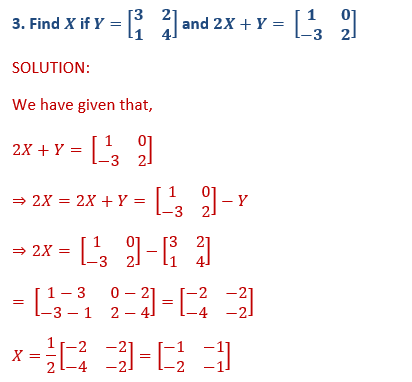# ML Aggarwal Solutions Class 10 Maths Chapter 8 Matrices

Exercise 8.1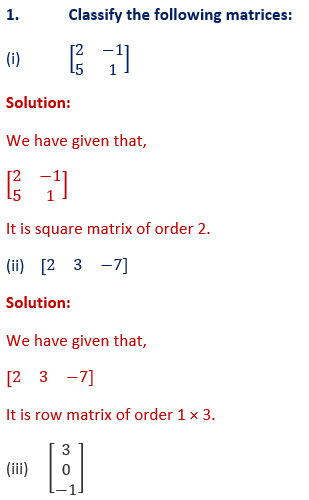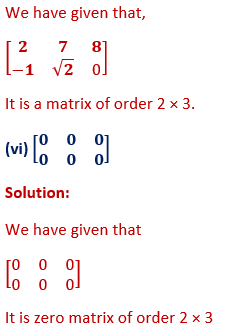2. (i) If a matrix has 4 elements, what are the possible order it can have?
Solution:
We have given that,
Matrix has 4 elements
It can have 1 × 4,4 × 1 or 2 × 2 order.

(ii) If a matrix has 4 elements, what are the possible orders it can have?
Solution:
We have given that,
Matrix has 4 elements,
It can have 1 × 8, 8 × 1, 2 × 4 or 4 × 2 order.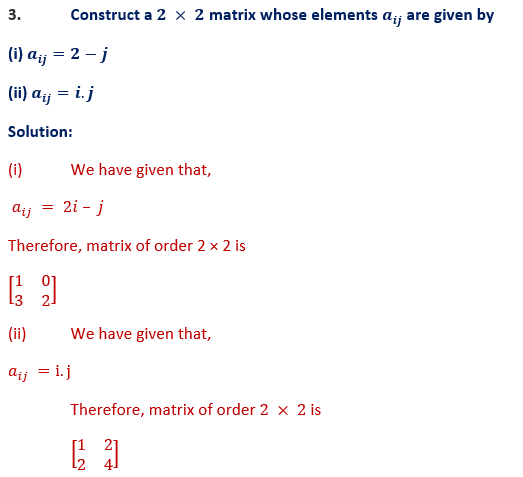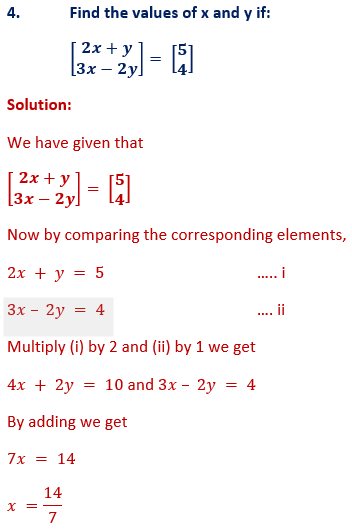x = 2
Substituting the value of x in (i)
4 + y = 5
y = 5 – 4
y = 1
Hence x = 2 and y = 1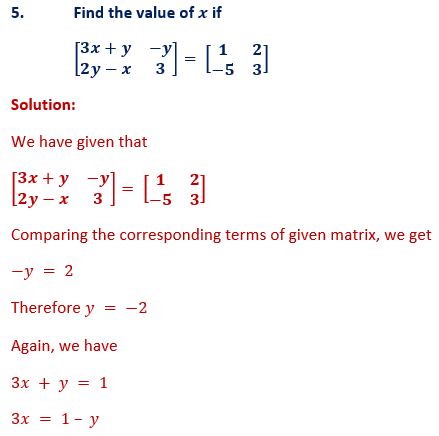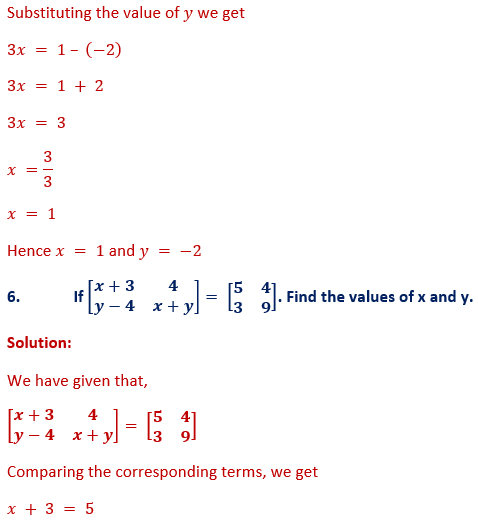x = 5 – 3
x = 2
Again we have
y – 4 = 3
y = 3 + 4
y = 7
Hence x = 2 and y = 7x + 2 = -5
x = -5 – 2
x = -7
Also, we have 5z = -20
z = -20/5
z = – 4
Again from given matrix we have
y2  + y – 6 = 0
The above equation can be written as
y2  + 3y – 2y – 6 = 0
y (y + 3) – 2 (y + 3) = 0
y + 3 = 0 or y – 2 = 0
y = -3 or y = 2
Hence x = -7,y = -3,2 and z = -4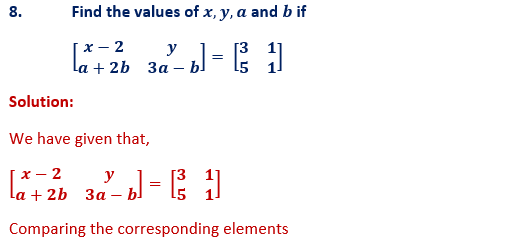x – 2 = 3 and y = 1
x = 2 + 3
x = 5
Again, we have
a + 2b = 5 ... i
3a – b = 1 …ii
Multiply (i) by 1 and (ii) by 2
a + 2b = 5
6a – 2b = 2
Now by adding above equations we get
7a = 7
a =7/7
a = 1
Substituting the value of a in (i) we get
1 + 2b = 5
2b = 5 -1
2b = 4
b =4/2
b = 2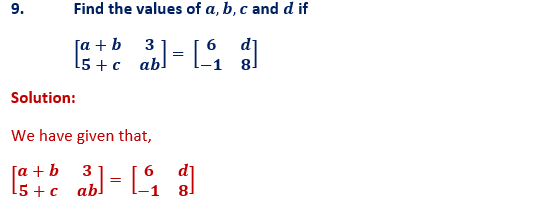Comparing the corresponding terms, we get
3 = d
d = 3
Also we have
5 + c = -1
c = -1 – 5
c = -6
Also we have,
a + b = 6 and a b = 8
We know that,
(a – b)2  = (a + b)2  – 4ab
(6)2  – 32 = 36 – 32 = 4 = (± 2)2
a – b = ± 2
If a – b = 2
a + b = 6
Adding the above two equations we get
2a = 4
a =4/2
a = 2
b = 6 – 4
b = 2
Again, we have a – b = -2

and a + b = 6
2a = 4
a =4/2
a = 2
Also, b = 6 – 2 = 4
a = 2 and b = 4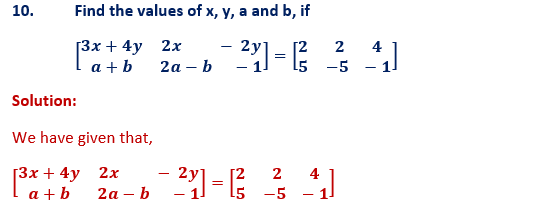Comparing the corresponding terms, we get
3x + 4y = 2 ….i
x – 2y = 4 …..ii
Multiply (i) by 1 and (ii) by 2
3x + 4y = 2
2x – 4y = 8
Adding the above equations we get
5x = 10
x =10/5
x = 2
Substituting the value of x in (i)
We get
6 + 4y = 2
4y = 2 – 6
4y = -4
y = -1

Exercise 8.2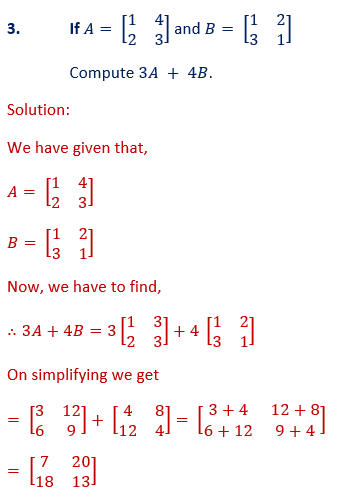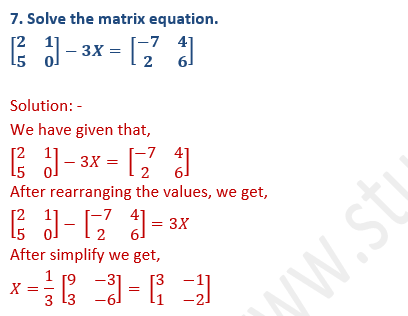EXERCISE : 8.3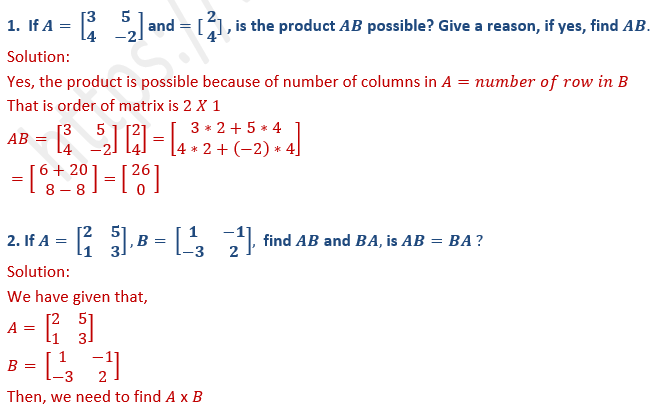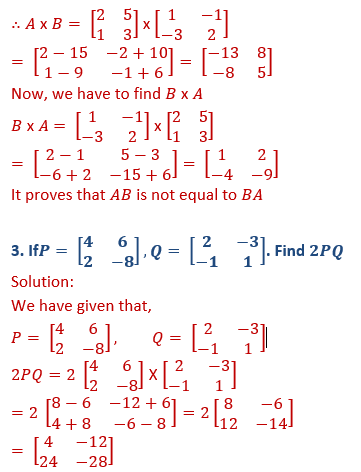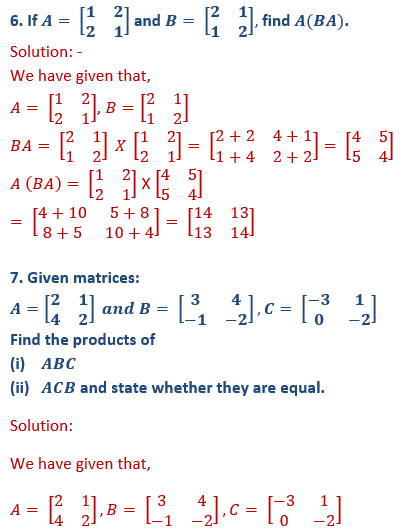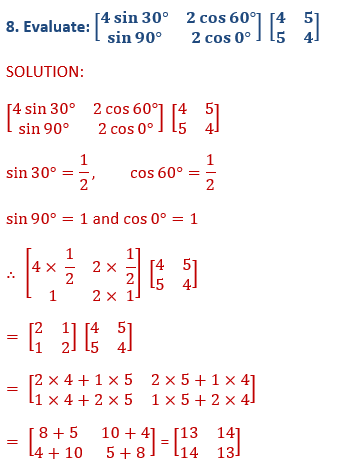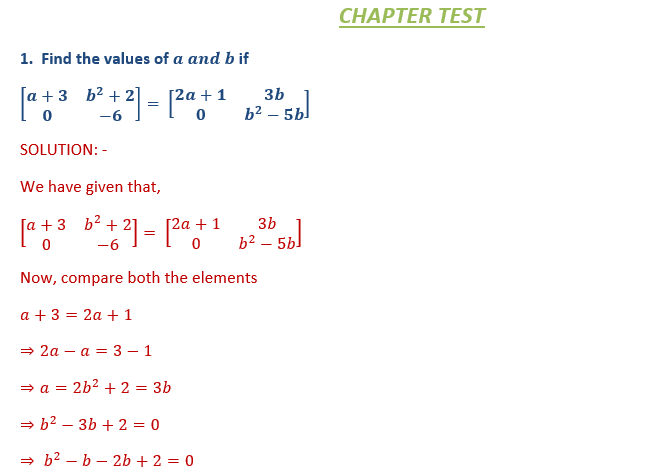⇒b(b-1)-2(b-1)=0
⇒(b-1)(b-2)=0
Either b-1=0,
Then, b=1 or b-2=0,
Then, b=2
Hence a=2,b=2 or 1Now, compare both the elements
3a=4+a
a-a=4
2a=4
∴ a=2
3b=a+b+6
3b-b=2+6
2b=8
∴b=4
3d=3+2d
3d-2d=3
∴d=3
3c=c+d-1
3c-c=3-1
2c=2
∴c=1
Hence a=2,b=4,c=1 and d=3